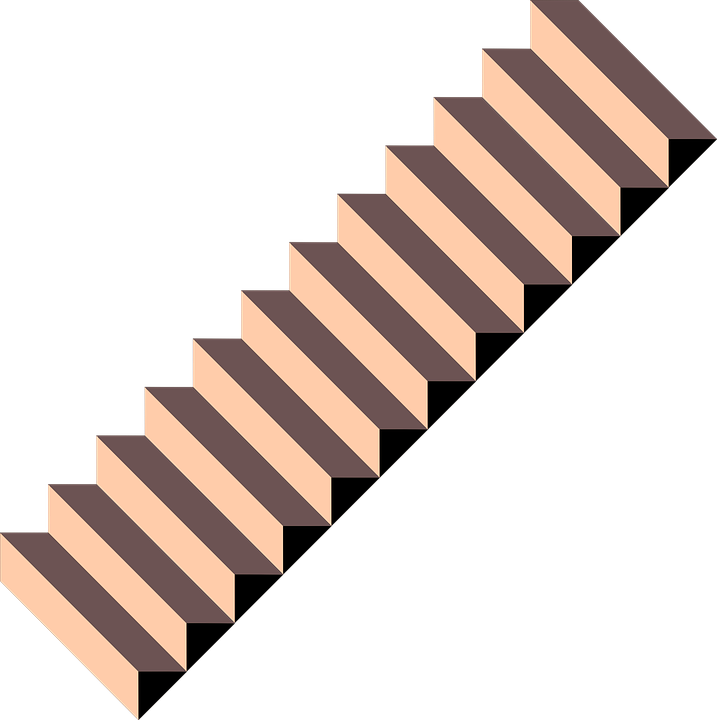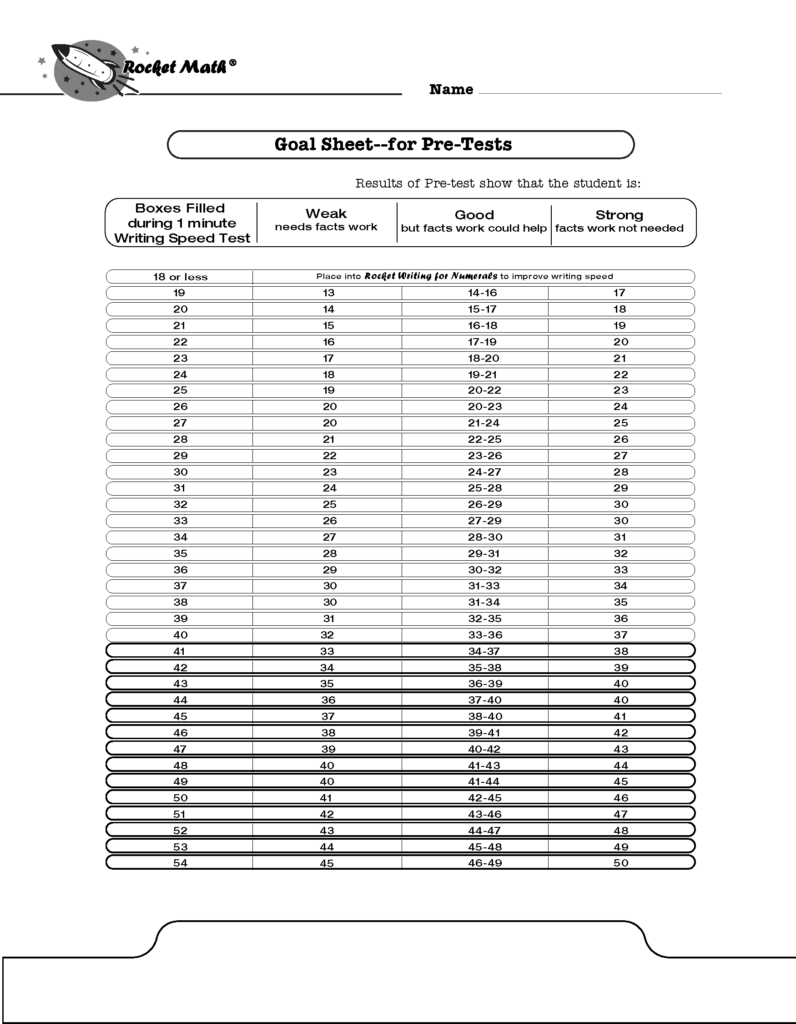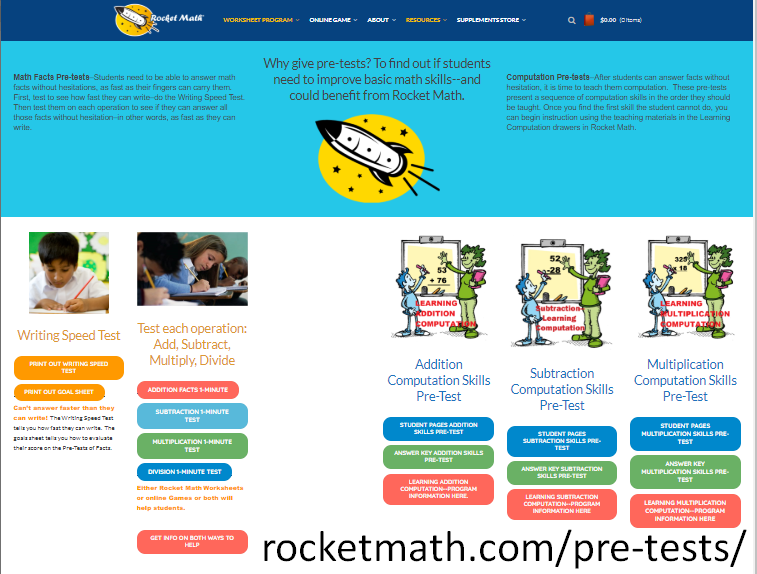## Math Fact Benchmarks: Why Writing Speed Matters

Math drills are used to help students learn their basic single-digit math facts. The goal is to help students answer basic math facts from recall and develop automaticity. The only way to tell if a student is recalling the answer rather than figuring it out is by using timed math drills as benchmarks. But students cannot learn how to recall a full page of math facts by drilling on them. The drills will likely cause anxiety and frustration for students. Here are some common pitfalls that are wise to avoid when teaching students basic single-digit facts.

## Common Problems With Math Benchmarks

Schools typically set “benchmarks” to evaluate whether students have achieved their educational goals.  When it comes to math fact memorization, schools will set benchmarks to distinguish between knowing the answers by a quick recall from the slow process of figuring out the facts. There are two main problems with these benchmarks.### Learned one at a time.

The first problem is that math facts are learned individually and students meet the benchmark one problem at a time. The benchmark should be to answer a problem in less than a second. If they can, then it would be clear which math facts a student has memorized and which ones they don’t. It is more common for schools to give tests on all the facts in an operation and these tests can’t tell the schools which facts are memorized. A more meaningful report would be which facts in an operation can a student answer instantly.### Time to write the answer.

The second main problem is that no matter how well students know facts, they cannot write answers to facts any faster than they can write. Elementary students vary in speed and can write answers anywhere from 10 answers in a minute to 50 in a minute. If a student can answer 40 problems in a minute, they have achieved mastery of math facts. But if they can’t write answers that quickly, they can not meet their school’s benchmark. In order to set a reasonable standard, teachers need to know how fast students can write and a writing speed test will help determine this.

## Common Core Math Fact Fluency Need Not Cause Anguish

The Common Core Idea says that students must have a quick recall of math facts to progress successfully in math. For some reason, almost all children have memorized 2+2=4 and can answer this problem from recall. It is not stressful or hard and will not cause math phobia. So, we know that learning how to answer a math problem instantly from recall is doable and can be done without being stressful. The challenge is that there are a lot of facts to learn, but taken a few at a time, they can all be learned equally easily.

To do this though, it takes a systematic effort, daily practice, and careful monitoring. Teachers need a facts program of some kind to help their students commit a large number of facts to memory. Before developing the design principles behind Rocket Math, I had thought this was a nearly impossible task. But in the last 20 years, I have seen how Rocket Math has been used successfully to teach math facts to students. With plenty of time, patience, and encouragement, students can learn to recall math facts from memory and with Rocket Math they can do this while having fun.

## Rocket Math Writing Speed Test

Here is a simple easy-to-use Writing Speed Test to help figure out how fast a student is able to answer when they have all the facts in an operation memorized. The test is a mix of one and two-digit numbers so it works with addition and multiplication.

Simply give the test to your students and have them write the numbers they see in each box for one minute. You will be able to find out how many boxes they can complete in one minute. That number is the upper limit of math fact problems you can expect them to be able to answer–if they are answering from recall rather than by figuring them out.

### Writing speed is number of boxes completed.

When the student has finished the test, you will have the number of boxes they can complete in a minute. You can expect the student to be able to finish at least 80% of that number if they can recall the facts instantly.  If the student can answer 90%, they don’t need any fact work. If they are between 80 and 90% of that number, they are good, but more facts work would help them. Anything below 80% and they are having to stop to figure out some of the facts and need more work.If you’re not interested in doing the math over and over, here is a goal sheet for pre-tests, where the numbers are worked out for you. You can print it from this link.

### Benchmarks must be based on writing speed.

Benchmarks that don’t take into account the speed at which children can write, leave a lot of children with an impossible expectation. Asking children to do something they are physically incapable of doing will cause a lot of unnecessary anguish.

## Kindergarten Math BenchmarksStudents should be taught how to write numerals correctly and efficiently in kindergarten. The methods of drawing numerals that children invent on their own can be slow, cumbersome, and inefficient – causing them to have a slow writing speed later. Rocket Math Writing for Numerals is a systematic method that will help students learn how to form their numerals. Benchmarks for kindergarteners should focus on the speed of writing numerals, which takes a good amount of practice and instruction.

Kindergarten Numeral Writing Fluency Benchmarks (digits)

• Mid-year
• 20 digits per minute
• End of year
• 40 digits /minuteFirst-grade students need to be fluent in writing numerals. Not every kindergarten does this, so teachers should test and give some kind of numeral writing program to students who do not meet these writing standards.

First Grade Numeral Writing Fluency Benchmarks (digits)

• Start of year
• 40 digits per minute
•  Mid-year
• 60 digits per minute
•  End of year
• 60 digits per minute

We also want first-graders to learn addition facts to the level of instant recall. The student’s writing speed is the number of boxes they can complete in one minute.

First Grade Math Fact Fluency Benchmarks (problems)

• Start of year
• Addition 20% of writing speed
•  Mid-year
• Addition 40% of writing speed
• End of year
• Addition 80% of writing speed
• Subtraction 20% of writing speed

In second-grade we want students to master subtraction facts as well as addition.

• Start of year
• Addition 80% of writing speed
• Mid-year
• Addition 80% of writing speed
• Subtraction 40% of writing speed
• End of Year
• Addition 80% of writing speed
• Subtraction 80% of writing speedIn third-grade it is important that students master subtraction and begin working on multiplication.

• Start of year
• Addition 80% of writing speed
• Subtraction 80% of writing speed
• Mid-year
• Addition 80% of writing speed
• Subtraction 80% of writing speed
• Multiplication 40% of writing speed
• End of year
• Addition 80% of writing speed
• Subtraction 80% of writing speed
• Multiplication 80% of writing speed

In fourth-grade it is important for the students to have multiplication mastered and begin division.

• Start of the Year
• Addition 80% of writing speed
• Subtraction 80% of writing speed
• Multiplication 80% of writing speed
• Mid-year
• Addition 80% of writing speed
• Subtraction 80% of writing speed
• Multiplication 80% of writing speed
• Division 40% of writing speed
• End of year
• Addition 80% of writing speed
• Subtraction 80% of writing speed
• Multiplication 80% of writing speed
• Division 80% of writing speed

## Fifth-grade (and up) math fact benchmarksIn fifth-grade it is important for the students to have mastered their basic math facts in addition, subtraction, multiplication, and division.

• Start of year
• Addition 80% of writing speed
• Subtraction 80% of writing speed
• Multiplication 80% of writing speed
• Division 80% of writing speed
•
•
• Mid-year
•  Addition 80% of writing speed
• Subtraction 80% of writing speed
• Multiplication 80% of writing speed
• Division 80% of writing speed
• End of year
• Addition 80% of writing speed
• Subtraction 80% of writing speed
• Multiplication 80% of writing speed
• Division 80% of writing speed

All the 1-minute pre-tests and the writing speed tests and the goal sheet can be found on this page https://rocketmath.com/pre-tests/.## Rocket Math Is A Fun Way For Students To Learn

Rocket Math has a systematic approach to teaching students how to become fluent in basic math facts and still have fun learning math. Math can seem daunting to learn and if not taught properly, using a good math program, students can build a math phobia. Teachers have been using Rocket Math for over 20 years now and believe it to be an invaluable tool to help their students learn math.